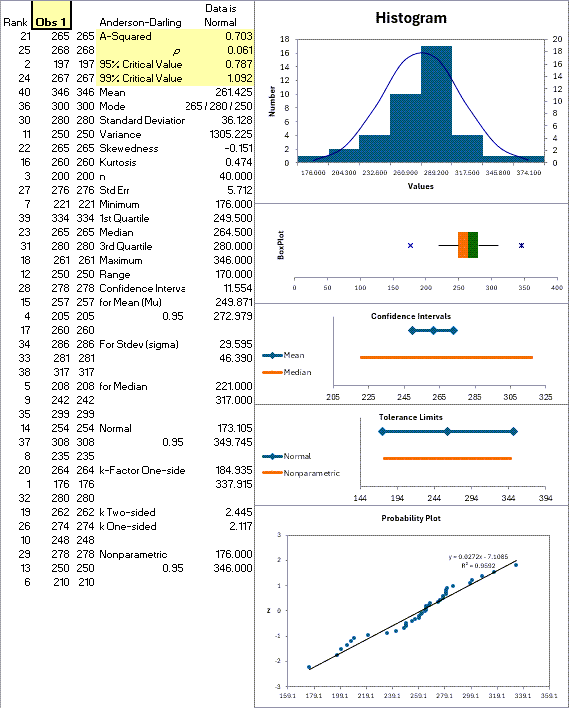# Descriptive Statistics

Many customers call and ask if Excel or the QI Macros Statistical Software can calculate descriptive statistics on their data like standard deviation, variance and confidence intervals. The answer is yes.

### QI Macros Descriptive Statistics

QI Macros descriptive statistics is much easier to use than Excel's and we have added a few additional calculations and charts of our own. Just click and drag over your data to select it, then click on QI Macros, Statistical Tools, Descriptive Statistics.QI Macros output contains all of the descriptive statistics Excel includes in it's output plus it also contains:

• A data normality test (Anderson Darling Test) and
• Charts: histogram, box plot, capability plot and normal probability plot:
•### Excel's Descriptive Statistics

Excel can calculate some descriptive statistics for your data, but its interfaces are difficult to understand. In Excel click on Tools, Data Analysis and Descriptive Statistics to get this input window:Excel calculates a separate set of statistics for each column or row of your data. If you want to calculate statistics for all of your data, make sure your data is only in one row or column. Excel's output looks like this:Show less

### Excel's Function Key

You can use Excel's function key (fx) to calculate some statistics:However, you may have to perform some preliminary calculations on your own and set up inputs of the calculation in a spreadsheet. For example, if you wanted to calculate confidence intervals for a set of data you would need to perform the following steps:

• Calculate n, average, standard deviation and alpha for your data.
• Input these values into a spreadsheet, then label a cell "Confidence Interval" and click on the fx key to insert a function into the cell formula:• Click okay and define the inputs.
• Show less

Save yourself time and frustration, use QI Macros Statistical tools for your statistical needs.

### Why Choose QI Macros Statistical Software for Excel?### Easy to Use

• Works Right in Excel
• Interprets p-values for You
• Accurate No-Worry Results
• Free Training Anytime### Proven and Trusted

• 100,000 Users in 80 Countries
• Celebrating 20th Anniversary
• Five Star CNET Rating - Virus Free### Affordable

• Only \$349 USD
Quantity Discounts Available
• No annual fees
• Free Technical Support